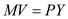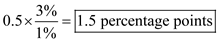# Quiz 19: Current Issues in Macro Theory and Policy

Business

The basic equation of monetarism is:Where: • M denote money supply , • V denote money velocity , • P denote price level , and • Y denotes real GDP. The left-hand-side of the equation is the total money in circulation, and the right-hand-side of the equation is the dollar value of output, or the total money demanded. The supply and demand of money must equal. Given: • Money supply for a Hypothetical economy is \$96 billion. • The nominal GDP for a Hypothetical economy is \$336 billion. Now, substitute the given values in the formula:The velocity iS3.5 times per year, meaning thaT1 dollar circulatE3.5 times a year. In the short run, the decrease of money supply will bring down aggregate demand and real output. In the long run, wages come down and firms increase real output. The aggregate supply curve shifts to the right; real output goes back to its full employment level and price level drops. Thus, nominal GDP falls by \$20 billion , the same amount as money supply.

If the prices are fixed, the aggregate supply curve is a horizontal line. If AD decreases in this situation, equilibrium price stays the same and equilibrium output decreases. When output price is flexible, the aggregate supply curve is upward-sloping. If AD decreases in this situation, both equilibrium price and equilibrium output increase. When both input price and output price are flexible, the aggregate supply curve is a vertical line. If AD decreases in this situation, equilibrium price increases and equilibrium output stays the same at the full-employment level.

The basic equation of monetarism isWhere: • M denote money supply , • V denote money velocity , • P denote price level , and • Y denotes real GDP. The left-hand-side of the equation is the total money in circulation, and the right-hand-side of the equation is the dollar value of output, or nominal GDP. a) The level of nominal GDP is calculated as follows:Thus, the level of nominal GDP in year 1 is \$160 billion. b) According to Taylor rule, when real GDP growth iS1% above potential GDP, the Fed should raise the real Federal fund rate by 0.5 percentage points. Since the annual growth of potential GDP iS3%, the Fed should raise the real Federal fund rate byThus, the fed should raise the real Federal fund rate by 1.5 percentage points. In order to obtain this goal the Fed need to buy securities from banks or the public between year 1 and 2 to meet its monetary rule.